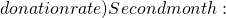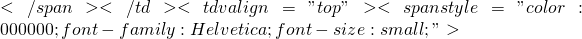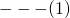# APGP Question #3This is a question from a recent ACJC JC2 test.

A philanthropist started a donation matching programme to encourage more people to donate regularly to a particular charity.

(a) For a person who donatesmore than the previous month, the philanthropist will donate20 in the third month, and ten times more than John in the seventh month.

(ii) Find the total amount of money donated by John and the philanthropist in one year, leaving your answer to the nearest dollar.

(b) In a revised donation matching programme, if a donor makes a monthly donation oflatex 0\%latex 10 \%latex 20 \%latex 30 \%latex 4^{th}2c.

(ii) By expressing the total amount of money, the philanthropist will donate at the end of themonth as a summation, and using the result, show that the total amount of money the philanthropist will donate at the end of themonth is latex \frac{(n-1)n(n+1)}{30} clatex n^{th}latex alatex alatex a + b^2latex ablatex a + 6b^2latex ab^6latex ab^2 = 20latex ab^6 = 10(a+ 6b^2)latex b^2 = \frac{20}{a}latex \Rightarrow a(\frac{20}{a})^3 = 10[a + 6(\frac{20}{a})]latex a^3 + 120a – 800 = 0latex a = 5.373610646 \approx 5.37 (3 SF)latex b = 1.929220642 \approx 1.93 (3 SF)latex = \frac{12}{2} [2(5.373610646) + (12-1)(1.929220642)^2] = 310.1282186latex = \frac{5.373610646(1.929220642^{12} – 1)}{1.929220642 – 1} = 15366.20901latex = 15366.20901 + 310.1282186 = 15676.33723 \approx 15676.34\$

[/showhide]
Not readable? Change text.